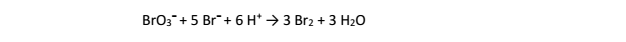# Problem: The balanced equation for the reaction of bromate ion with bromide in acidic solution is given by: At a particular instant in time, the value of −Δ[Br -]/Δt is 2.0 x 10-3 mol/L s. What is the value of Δ[Br2]/Δt in the same units? A. 1.2 x 10-3 B. 6.0 x 10-3 C. 3.3 x 10-3 D. 3.3 x 10-5 E. 2.0 x 10-3

🤓 Based on our data, we think this question is relevant for Professor Ratliff's class at USF.

###### Problem Details

The balanced equation for the reaction of bromate ion with bromide in acidic solution is given by:

At a particular instant in time, the value of −Δ[Br -]/Δt is 2.0 x 10-3 mol/L s. What is the value of Δ[Br2]/Δt in the same units?

A. 1.2 x 10-3

B. 6.0 x 10-3

C. 3.3 x 10-3

D. 3.3 x 10-5

E. 2.0 x 10-3What scientific concept do you need to know in order to solve this problem?

Our tutors have indicated that to solve this problem you will need to apply the Average Rate of Reaction concept. You can view video lessons to learn Average Rate of Reaction. Or if you need more Average Rate of Reaction practice, you can also practice Average Rate of Reaction practice problems.

What is the difficulty of this problem?

Our tutors rated the difficulty ofThe balanced equation for the reaction of bromate ion with b...as medium difficulty.

How long does this problem take to solve?

Our expert Chemistry tutor, Dasha took 2 minutes and 53 seconds to solve this problem. You can follow their steps in the video explanation above.

What professor is this problem relevant for?

Based on our data, we think this problem is relevant for Professor Ratliff's class at USF.